# Germanium¶

This document is part of the QuantumWise Semiconductor Whitepapers. Here we investigate computational methods for ATK-DFT calculations for bulk germanium in the diamond crystal structure. We apply the SG15 pseudopotentials and a number of exchange-correlation functionals; PBE, PBEsol, TB09-MGGA, and PBE with the Pseudopotential Projector-Shift method. A limited number of HSE calculatios have been done for comparison. See the section Introduction for more information.

The following quantities have been calculated for each computational method, both at the experimental germanium lattice constant and at the theoretically predicted ones:

• Elastic constants: Bulk modulus, Poisson ratio, and Young’s modulus.
• Band structure along the X–Γ–L Brillouin zone path.
• Band gaps: Γ–L (fundamental gap), Γ–Γ (direct gap), and the Γ–X and Γ–$$\Delta$$ indirect gaps.
• Electron effective masses in the L point; transverse and longitudinal.
• Static dielectric constant.

Convergence studies indicate that a 100 Hartree mesh cutoff in conjunction with a 8x8x8 k-point grid for calculation of elastic properties (e.g. lattice constant) and a 9x9x9 k-point grid for electronic properties (e.g. band gap) are suitable for well-converged and computationally efficient ATK-DFT calculations for bulk germanium. These settings were used for computing the quantities listed above.

The projector shifts used for the pps-PBE method are 15.0 eV for s-orbitals, 0.2 eV for p-orbitals, and $$-$$2.0 eV for d-orbitals. This yields a single computational method with fairly high accuracy for predicting the fundamental band gap and lattice constant for germanium. Note that these PPS parameters should only be used with SG15 pseudopotentials.

This document is organized as follows. The section Summary gives a short summary of the main results from this study, while the section Convergence briefly presents the convergence studies mentioned above, and the section Timing compares CPU timings and scalability of different SG15 basis sets for germanium. Next, the section Results contains figures illustrating the performance of the different computational methods in predicting the materials properties listed above. Finally, the Appendix gives a template QuantumATK Python script for setting up ATK-DFT calculations for germanium similar to the ones presented here.

## Summary¶

The following list summarizes the main conclusions from this study.

• The PBEsol, pps-PBE, and HSE methods all yield fairly accurate germanium lattice constants, but pps-PBE and HSE appear somewhat more accurate than PBEsol for computing elastic properties of germanium.
• GGA methods, such as PBE and PBEsol, tend to yield a qualitatively wrong (semi-metallic) germanium band structure. The TB09-MGGA, pps-PBE, and HSE methods do much better, though the lowest TB09-MGGA valence bands are in general shifted upwards as compared to those found with pps-PBE and HSE. Note, however, that the TB09-MGGA c-parameter was computed self-consistently. Manually tuning the c-parameter could probably bring the TB09-MGGA valence bands down in energy.
• The PBE and PBEsol density functionals should not be used for computing germanium band gaps. Conversely, the pps-PBE and HSE methods can yield fairly accurate numbers for the fundamental band gap. Tuning the TB09-MGGA c-parameter may possibly bring better TB09-MGGA band gaps, but this was not tested.
• The predicted band gaps appear to depend somewhat on the germanium lattice constant used for the calculation. In general, the fundamental band gap increases as the unit cell volume decreases.
• Even though PBE and PBEsol do not predict the correct band gap ordering in germanium, they are actually significantly better for computing effective electron masses in the germanium L point (the experimental conduction band minimum), than the TB09-MGGA and pps-PBE methods. However, all tested methods overestimate the electron effective masses, especially the fairly small transverse effective mass.
• The PBE and PBEsol functionals should not be used for computing optical properties of germanium, such as the static dielectric constant. The vanishing Γ-point direct band gap with GGA methods is most likely the reason for this. The ppsPBE method appears significantly better in this respect.

## Convergence¶

The type of Monkhorst–Pack k-point grid and the density mesh cutoff energy are essential ATK-DFT parameters that affect the computational efficiency and precision: A dense k-point grid and high cutoff energy usually give high precision, but may also be computationally intense. It is therefore important to investigate the trade-off between computational precision and cost. Fig. 181 illustrates how the germanium total energy, fundamental band gap, and lattice constant depend on the mesh cutoff and k-point grid.Fig. 181 Convergence of the germanium total energy, fundamental (indirect) band gap, and lattice constant with respect to the ATK-DFT density mesh cutoff (top x-axis in all three panels; green and black dashed lines) and k-point grid (bottom x-axis in all three panels; blue and red lines). The ‘centered’ k-point grids are shifted to the $$\Gamma$$ point (only affects even grids, e.g. 4x4x4), while the ‘non-centered’ k-point grids are shifted by (0.5,0.5,0.5) and therefore do not sample the $$\Gamma$$ point (only affects odd grids, e.g. 3x3x3). The horizontal dotted lines indicate tight convergence criteria.

Note that a standard Monkhorst–Pack k-point grid is Γ-centered for odd numbers of points (e.g. 3x3x3), but does not include the Γ point for even number of points (e.g. 4x4x4). The blue lines in Fig. 181 are for k-point grids where even grids are shifted to Γ (such that both 3x3x3 and 4x4x4 grids do include Γ), while the red lines are for calculations where odd grids are shifted away from Γ (such that both 3x3x3 and 4x4x4 grids do not include Γ).

For the SG15 pseudopotential with both Medium and High basis sets, a 100 Hartree mesh cutoff gives band gaps and lattice constants that are converged to within 10-4 eV and roughly 10-4 Å, respectively.

It is also clear that an off-Γ k-point grid (non-centered, red) gives faster convergence of the total energy and lattice constant than the k-point grid that includes the Γ point (centered, blue). We see that the non-centered 8x8x8 Monkhorst–Pack grid yields higly converged lattice constants.

On the other hand, the computed band gap converges fastest with the Γ-centered k-point grid (blue). This may be because the valence band maximum is located at the Γ point. The 9x9x9 Monkhorst–Pack grid is Γ-centered by default, and yields highly converged band gaps.

Note

All remaining ATK-DFT calculations in this study therefore use a 100 Hartree mesh cutoff energy and a standard (non-centered) 8x8x8 Monkhorst–Pack k-point grid for elastic properties and the standard 9x9x9 grid for computing electronic properties.

## Timing¶

The computational cost of a calculation may depend critically on the choice of basis set, particularly for calculations on large systems with many atoms. Fig. 182 shown below illustrates this for the present case of germanium and the Medium, High, and Ultra SG15 basis sets.

The germanium primitive cell (containing 2 atoms) was systematically repeated in steps of one along all three lattice vectors, resulting in rapidly increasing systems sizes. PBE calculations were then performed for each system, using Γ-point sampling only, to avoid any influence of k-point sampling on the recorded CPU times.

It is quite clear from the figure that the computational loads of the SG15 Medium and High basis sets for germanium are not much different for relatively small system sizes, but the High basis set becomes more demanding for larger system sizes. Moreover, the Ultra basis set appears in comparison extremely demanding, and will not be used in the remaining calculations in this study.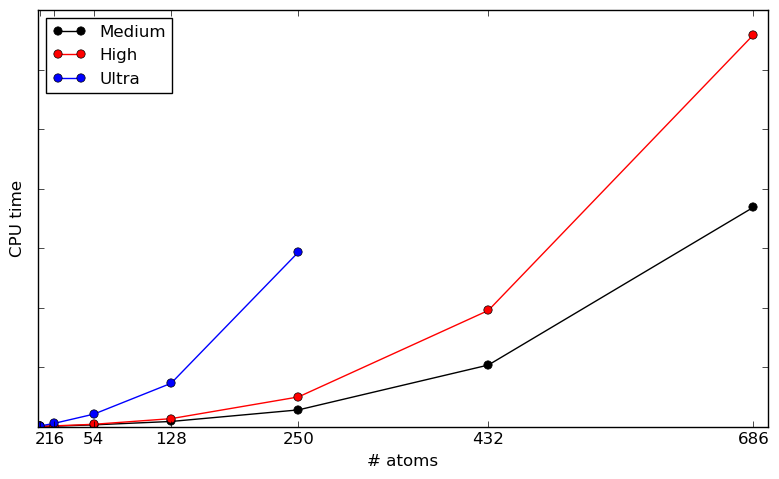Fig. 182 Total CPU time for the first 5 SCF iterations by the ATK-DFT calculator for the Medium (black), High (red), and Ultra (blue) SG15 basis sets, against the number of germanium atoms in the increasingly larger (repeated) bulk unit cell. Only the Γ point is sampled (1x1x1 k-point grid) and the calculations were executed on a single CPU core. Note that both figure axes are linear. For reference, the 432-atom SG15-Medium calculation reach full convergence in 11 SCF steps, which take less than 30 minutes if running in parallel on 16 modern CPU cores.

## Results¶

This section presents ATK-DFT results obtained with the PBE, PBEsol, pps-PBE, and TB09-MGGA methods using Medium and High basis sets with the SG15 pseudopotential for germanium, as well as HSE results obtained with the FHI-aims calculator. The list below gives direct links to all subsections:

### Lattice constant¶

The PBEsol lattice constant for germanium is very close to the experimental 300 K value, 5.6574 Å, but the pps-PBE and HSE methods are also quite accurate. The bare PBE functional (without any pseudopotential projector shifts) is known to overestimate lattice constants in general, and for germanium by almost 3%.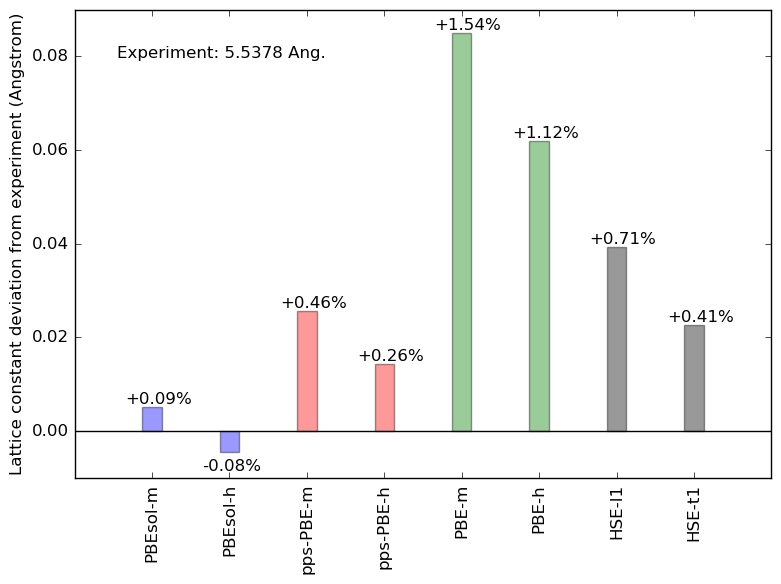Fig. 183 Germanium lattice constant calculated using the PBEsol (blue), pps-PBE (red), PBE (green), and HSE (black) methods, plotted as deviation from the experimental value.

### Elastic constants¶

The experimental bulk modulus, Poisson ratio, and Young’s modulus of germanium are well reproduced by the pps-PBE method, but HSE also does well in this respect. PBEsol is in general a bit more off, and pure PBE underestimates all three elastic quantities.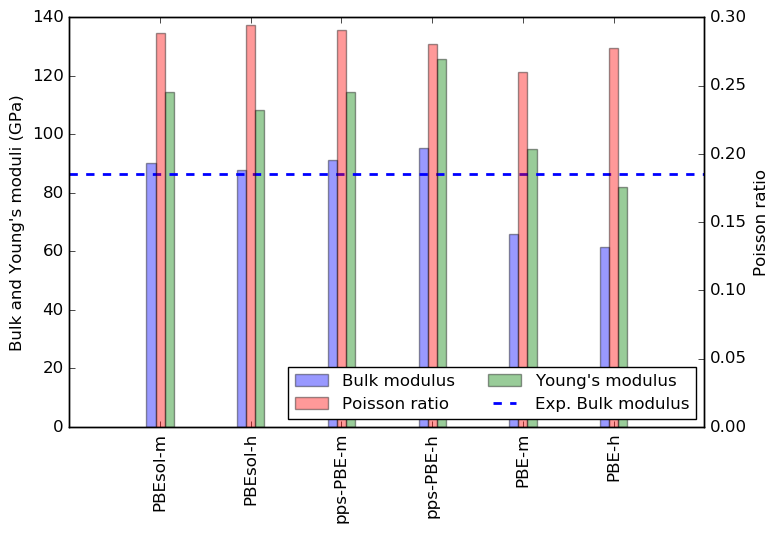Fig. 184 Germanium bulk modulus (blue), Poisson ratio (red), and Young’s modulus (green) calculated using the PBEsol, pps-PBE, PBE, and HSE methods. The blue dashed, red dotted, and green dash-dotted lines indicate the experimental values, all from www.crystran.co.uk.

### Band structure¶

The germanium fundamental band gap is fairly small (0.66 eV at 300 K), and occurs between the Γ and L high-symmetry points in the Brillouin zone. The remaining conduction band minima are quite close in energy (see also Band gaps). This band structure is hard to correctly reproduce within GGA-DFT. As seen in the figure below, PBE and PBEsol tend to close the direct gap at Γ, and yield a rather small Γ–L band gap. On the other hand, the TB09-MGGA, pps-PBE, and HSE methods correctly yield a significant direct band gap at Γ, and also appear to preserve the correct band gap ordering. The SG15 High basis set (dash-dotted lines) gives in general slightly lower band energies than the Medium basis set does (full lines). A similar trend is observed for the tight and light accuracy settings for the FHI-aims HSE calculations.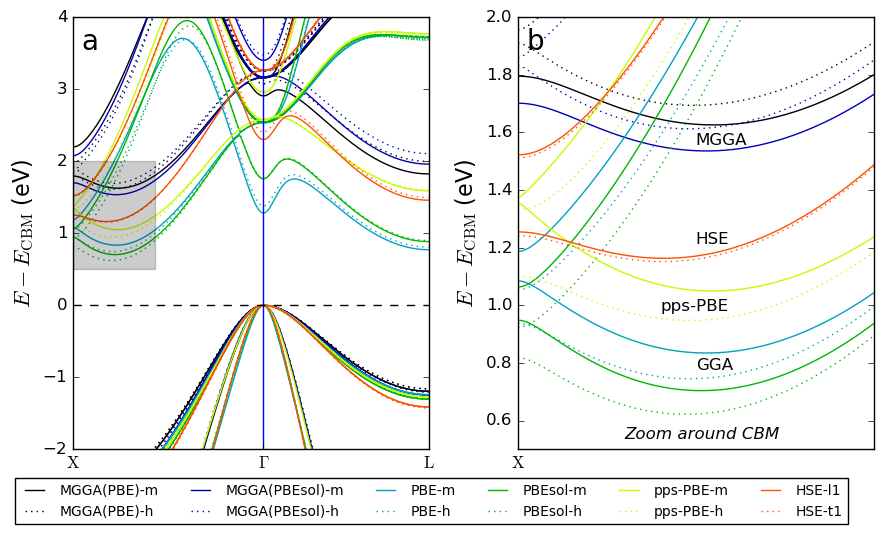Fig. 185 Germanium band structure calculated with the TB09-MGGA, PBE, PBEsol, pps-PBE, and HSE methods. All band structures are aligned at the $$\Gamma$$-point valence band maximum. The shaded area in panel a indicates the region around the conduction band minimum (CBM) shown in panel b.

### Band gaps¶

As noted just above, the PBE and PBEsol exchange-correlation functionals predict a qualitatively wrong band gap ordering for germanium; the Γ-point direct gap vanishes, and the lowest conduction band at the L point may even cross the Fermi level. No PBE or PBEsol band gaps are therefore included in the figure below.

The germanium band gaps are quite well reproduced by the pps-PBE and HSE methods. Especially the pps-PBE calculation at the pps-PBE lattice constant with the SG15 High basis set appears very accurate. The TB09-MGGA method correctly predicts that the fundamental germanium band gap is the Γ–L gap, but overestimates the band gaps in general. Note, however, that the TB09-MGGA c-parameter was calculated self-consistently from the germanium electronic structure (no fitting), while the pps-PBE projector shifts were essentially fitted to the germanium fundamental gap and lattice constant. On the other hand, the TB09-MGGA functional cannot be used for geometry optimization, which the pps-PBE method is well suited for.

We also observe that the band gaps may depend somewhat on the applied germanium lattice constant. For example, the pps-PBE $$E_{\Gamma_1}$$ gap is significantly larger at the experimental lattice constant than at the pps-PBE lattice constant.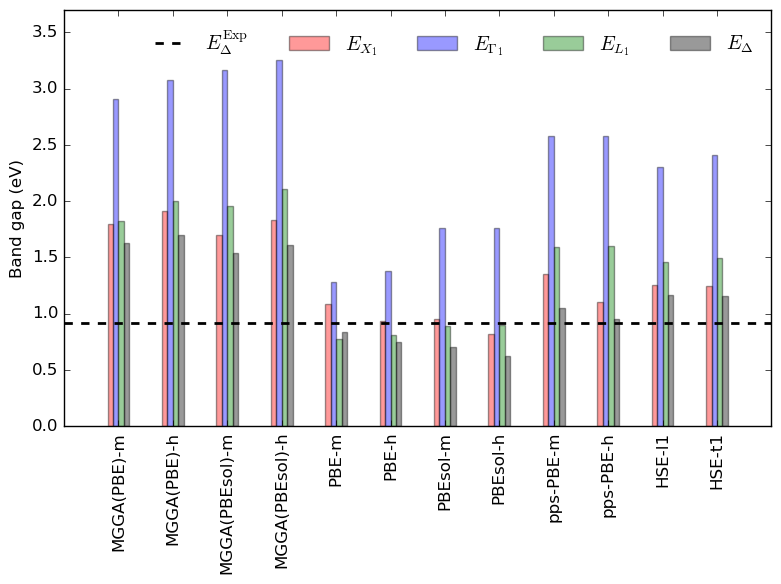Fig. 186 Germanium band gaps; $$E_\mathrm{g}$$ (fundamental gap, black) and energy gaps from the $$\Gamma$$-point valence band maximum to the conduction band minima at X (red), $$\Delta$$ (X-point valley, blue), and $$\Gamma$$ (green), calculated using the TB09-MGGA, pps-PBE, and HSE methods. Note that PBE and PBEsol calculations predict a qualitatively wrong band-gap ordering (often semi-metallic at $$\Gamma$$), so results from those methods are not included. TB09-MGGA at the PBE lattice constant yields a direct fundamental band gap, so TB09-MGGA(PBE) results are also not included. Experimental (300 K) values $$E_\mathrm{g}^\mathrm{Exp}$$ (black, dashed), $$E_{X_1}^\mathrm{Exp}$$ (red, dotted), $$E_\Delta^\mathrm{Exp}$$ (blue, dotted), and $$E_{\Gamma_1}^\mathrm{Exp}$$ (green, dotted) from http://www.ioffe.ru/SVA/NSM/Semicond/Ge/Figs/221.gif.

### Electron effective masses¶

The figure below shows the computed transverse (blue; left y-axis) and longitudinal (red; right y-axis) effective masses for electrons in the germanium conduction band minimum at the L point. PBE and PBEsol data are included, even though those methods do not capture that the conduction band minimum is at the L point. Both electron effective masses are in general overestimated by the TB09-MGGA and pps-PBE methods, while PBEsol and PBE do better, especially for the transverse effective masses.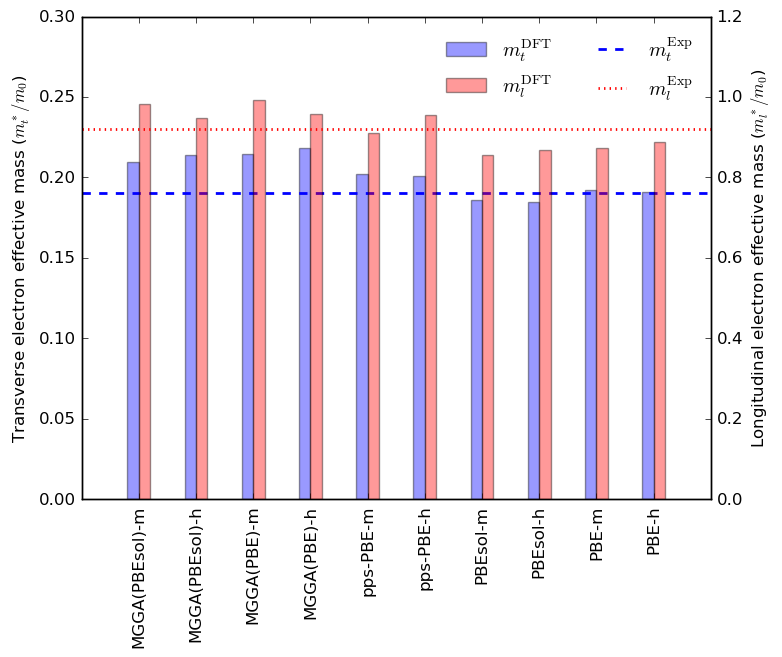Fig. 187 Germanium transverse (blue; left y-axis) and longitudinal (red; right y-axis) electron effective masses calculated in the L point (the experimental conduction band minimum), using the TB09-MGGA, pps-PBE, PBEsol, and PBE methods. The blue dashed and red dotted lines mark the experimental values, adapted from http://www.ioffe.ru/SVA/NSM/Semicond/Ge/bandstr.html.

### Dielectric constant¶

The static ($$\omega=0$$) dielectric constant of the germanium bulk is most reliably reproduced by the pps-PBE method: The pps-PBE values in the figure below are in general closer to experiment than the TB09-MGGA values, while the PBEsol and PBE values are far too large. The latter is most likely due to the completely wrong PBEsol and PBE germanium band structure in the region around the Fermi level.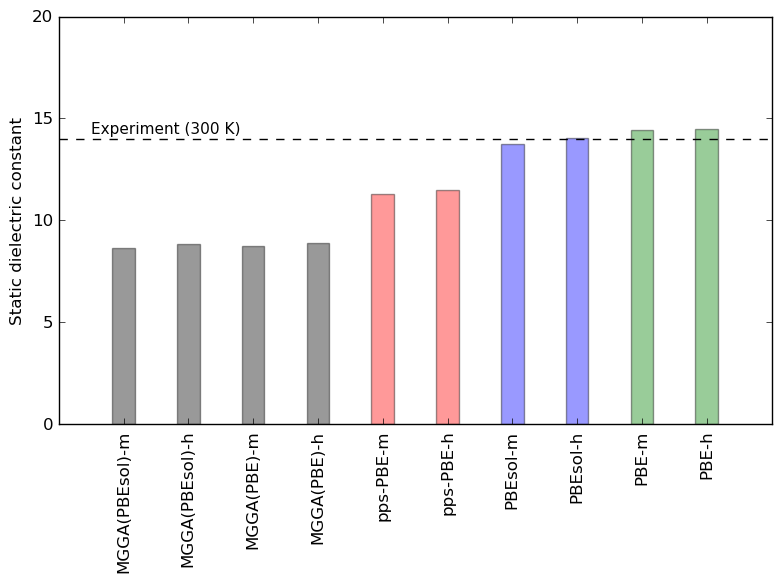Fig. 188 Static dielectric constant of germanium calculated using the TB09-MGGA (black), pps-PBE (red), PBEsol (blue), and PBE (green) methods. The number of electronic bands included in the OpticalSpectrum analysis were 10 below the Fermi level and 20 above it. The dashed line indicates the experimental value, 16.6, from www.crystran.co.uk.

## Appendix¶

The QuantumATK Python script shown below may be used as a template for ATK-DFT calculations for germanium with the SG15 pseudopotential. The script defines the germanium bulk configuration and then sets up the ATK-DFT calculator with PBE exchange-correlation. The script block named Basis Set shows various options for the SG15 basis set; ordinary PBE and PBE with pseudopotential projector shifts, both with Medium and High basis sets.

The script is available for direct download: germanium.py.

  1 2 3 4 5 6 7 8 9 10 11 12 13 14 15 16 17 18 19 20 21 22 23 24 25 26 27 28 29 30 31 32 33 34 35 36 37 38 39 40 41 42 43 44 45 46 47 48 49 50 51 52 53 54 55 56 57 58 59 60 61 62 63 64 65 66 67 68 # ------------------------------------------------------------- # Bulk Configuration # ------------------------------------------------------------- # Set up lattice lattice = FaceCenteredCubic(5.6574*Angstrom) # Define elements elements = [Germanium, Germanium] # Define coordinates fractional_coordinates = [[ 0. , 0. , 0. ], [ 0.25, 0.25, 0.25]] # Set up configuration bulk_configuration = BulkConfiguration( bravais_lattice=lattice, elements=elements, fractional_coordinates=fractional_coordinates ) # ------------------------------------------------------------- # Calculator # ------------------------------------------------------------- #---------------------------------------- # Basis Set #---------------------------------------- # Ordinary GGA: Medium or High basis set for SG15. basis_set = [BasisGGASG15.Germanium_Medium] #basis_set = [BasisGGASG15.Germanium_High] # GGA with pseudopotential projector-shift method: Medium or High basis set for SG15. #projector_shift = PseudoPotentialProjectorShift(s_orbital_shift=15.0*eV, # p_orbital_shift=0.2*eV, # d_orbital_shift=-2.0*eV) #basis_set = [BasisGGASG15.Germanium_Medium(projector_shift=projector_shift)] #basis_set = [BasisGGASG15.Germanium_High(projector_shift=projector_shift)] #---------------------------------------- # Exchange-Correlation #---------------------------------------- exchange_correlation = GGA.PBE k_point_sampling = MonkhorstPackGrid( na=9, nb=9, nc=9, ) numerical_accuracy_parameters = NumericalAccuracyParameters( k_point_sampling=k_point_sampling, density_mesh_cutoff=100.0*Hartree, ) iteration_control_parameters = IterationControlParameters( damping_factor=0.4, ) calculator = LCAOCalculator( basis_set=basis_set, exchange_correlation=exchange_correlation, numerical_accuracy_parameters=numerical_accuracy_parameters, iteration_control_parameters=iteration_control_parameters, ) bulk_configuration.setCalculator(calculator) nlprint(bulk_configuration) bulk_configuration.update() nlsave('Germanium.nc', bulk_configuration)